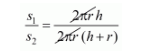# The ratio of lateral surface area to the total`
Question:

The ratio of lateral surface area to the total surface area of a cylinder with base diameter 1.6 m and height 20 cm is

(a) 1 : 7

(b) 1 : 5

(c) 7 : 1

(d) 8 : 1

Solution:

$r=\frac{1.6 \mathrm{~m}}{2}$

$=\frac{160}{2}$

$=80 \mathrm{~cm}$

$h=20 \mathrm{~cm}$

The ratio of lateral surface to the total surface area of cylinder$\frac{s_{1}}{s_{2}}=\frac{h}{(h+r)}$

$=\frac{20}{(20+80)}$

$\frac{s_{1}}{s_{2}}=\frac{20}{100}$

$=\frac{1}{5}$

$s_{1}: s_{2}=1: 5$

Hence, the correct answer is choice (b).